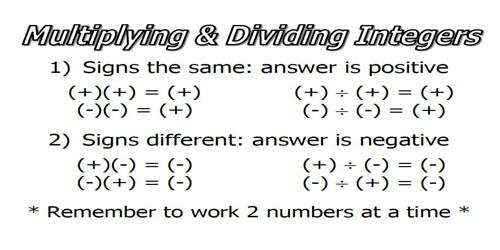Division of Integers: Negative and Positive Forms

Division of Integers is similar to division of whole numbers (both positive) except the sign of the quotient needs to be determined.

• RULE 1: The quotient of a positive integer and a negative integer is negative.
• RULE 2: The quotient of two positive integers is positive.
• RULE 3: The quotient of two negative integers is positive.

If both the dividend and divisor are positive, the quotient will be positive.

(+16) ÷ (+4) = +4

If both the dividend and divisor are negative, the quotient will be positive.

(-16) ÷ (-4) = +4

If only one of the dividend or divisor is negative, the quotient will be negative.

(+16) ÷ (-4) = -4     or      (-16) ÷ (+4) = -4

In other words, if the signs are the same the quotient will be positive, if they are different, the quotient will be negative.Explanation

Dividing positive and negative numbers follows the same rules as multiplying positive and negative numbers. We’ll repost them here for you, using division examples instead of multiplication:

Rule 1: A positive number divided by a positive number gives you a positive number.

Example 1: This is the kind of division you’ve been doing for years, positive numbers divided by positive numbers. It would look like this: 12/3 = 4. 12 is positive, 3 is positive, thus, 4 is positive. We know that 12 and 3 are both positive because there are no negative signs in front of them.

Rule 2: A positive number divided by a negative number gives you a negative number

Example 2: This is new – for example, you might have 12/-3. The 12 is positive, but the 3 is negative, so our answer has to be negative. Thus, we divide the numbers as we normally would, and then put a negative sign in front of our answer. So, 12/-3 = -4. Please note that this also works when the negative number comes first and the positive number is second. For example, you may see it written -12/3 = -4, but don’t get confused. The combination of one positive and one negative number, no matter which order they come in, means your answer is going to be negative.

Rule 3: A negative number divided by a negative number gives you a positive number.

Example 3: This is also new—and doesn’t seem to make much sense, but it is a rule we have to follow when dividing negative numbers. So, for example, we may have the problem -12/-4. Both the 12 and the 4 are negative, so we know our answer is going to be positive. Therefore, -12/-4 = 3.

Information  Source: